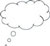# 3 Making connections

The first activity shows how division is important in everyday calculations and how the division algorithm can be made more understandable by using it in a context that the students may be familiar with. In mathematics, division is an important part of many topics. In fact, the big concept of proportionality, which incorporates fractions and ratio, relies on students’ understanding of division.

For example, a proportional division of a cake is one that gives each person at least a portion, which can be called a ‘proportional share’.

The next activity starts to extend the context of Activity 1 in order to help the students build their ideas of division.

## Activity 2: The meaning of a remainder

A box contains four tiles. Rajni has to calculate the number of boxes of tiles that she will need to buy to cover the floor.

• From the diagram that you drew in Activity 1, calculate the total area of the rectangle that represents the floor. How is that area related to the total area of the actual bedroom?
• What is the area that is covered by one tile of each kind?
• What is the total area that can be covered if Rajni buys one box of tiles of each kind?
• How many boxes will Rajni need in total?
• Will Rajni be able to completely use all the tiles she buys? How many tiles will remain unused? Is the remainder the same for both designs of tile, Pink Sparrow and Rosewood Matte?

## Case Study 2: Mrs Nagaraju reflects on using Activity 2

This was done the next day after doing Activity 1. I had collected the diagrams made by the students when we did Activity 1 so that they would not have to draw them again. I distributed the drawings back to the groups that they had worked in yesterday. Then I asked them to now work on the questions for this activity.

I reminded the groups that they would all have to be prepared to answer the questions I put up on the blackboard, so all of them should understand what the group is doing and not leave it to one or two to do all the calculations, and more importantly to know why they had done the calculations that they had.

Of course, they all had their carefully (or not so carefully) drawn diagrams from yesterday showing the tiles all laid out. Many groups started a discussion on whether they should just count the tiles drawn on their diagrams. Some of them did the counting and then the division and so both verified their answers, which showed they were really starting to understand the connections. But not all tried it by the same method.

It was an interesting discussion about how the remainder in both the cases was different and why that was. I also liked how the students linked ‘what remains’ to the word ‘remainder’ in the whole-class discussions and I noticed that in some of the group discussions they also used the words ‘what is left over’ and ‘leftover’ to describe this.Pause for thought What responses from students were unexpected? What did these responses tell you about their understanding of the meaning of a remainder?What points did you feel you had to reinforce?Did you modify the task in any way? If so, what was your reasoning for this?

2 Being able to see what is happening in division

4 The division algorithm again